# 2.2: Effect of Conjugation

•• Thomas Wenzel
• Bates College
$$\newcommand{\vecs}{\overset { \rightharpoonup} {\mathbf{#1}} }$$ $$\newcommand{\vecd}{\overset{-\!-\!\rightharpoonup}{\vphantom{a}\smash {#1}}}$$$$\newcommand{\id}{\mathrm{id}}$$ $$\newcommand{\Span}{\mathrm{span}}$$ $$\newcommand{\kernel}{\mathrm{null}\,}$$ $$\newcommand{\range}{\mathrm{range}\,}$$ $$\newcommand{\RealPart}{\mathrm{Re}}$$ $$\newcommand{\ImaginaryPart}{\mathrm{Im}}$$ $$\newcommand{\Argument}{\mathrm{Arg}}$$ $$\newcommand{\norm}{\| #1 \|}$$ $$\newcommand{\inner}{\langle #1, #2 \rangle}$$ $$\newcommand{\Span}{\mathrm{span}}$$ $$\newcommand{\id}{\mathrm{id}}$$ $$\newcommand{\Span}{\mathrm{span}}$$ $$\newcommand{\kernel}{\mathrm{null}\,}$$ $$\newcommand{\range}{\mathrm{range}\,}$$ $$\newcommand{\RealPart}{\mathrm{Re}}$$ $$\newcommand{\ImaginaryPart}{\mathrm{Im}}$$ $$\newcommand{\Argument}{\mathrm{Arg}}$$ $$\newcommand{\norm}{\| #1 \|}$$ $$\newcommand{\inner}{\langle #1, #2 \rangle}$$ $$\newcommand{\Span}{\mathrm{span}}$$$$\newcommand{\AA}{\unicode[.8,0]{x212B}}$$

## Compare the UV absorption spectrum of 1-butene to 1,3-butadiene.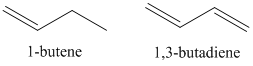Figure $$\PageIndex{8}$$ show the sign of the p-orbital wave functions (dark is positive, white is negative) used in creating the $$\pi$$ and $$\pi$$* orbitals. In the $$\pi$$-orbital, the two wave functions have the same sign and therefore positive overlap. In the $$\pi$$*-orbital, the two wave functions have the opposite sign and therefore negative overlap.Figure $$\PageIndex{8}$$. Representation of overlap of p-orbitals of a double bond.

In organic compounds, the bonding orbitals are almost always filled and the anti-bonding orbitals are almost always empty. The important consideration becomes the ordering of the molecular orbitals in an energy level diagram. Figure $$\PageIndex{9}$$ shows the typical ordering that would occur for an organic compound with $$\pi$$ orbitals.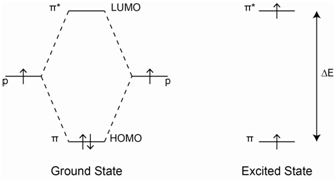Figure $$\PageIndex{9}$$. Energy level diagram for a compound with a $$\pi$$- and $$\pi$$*-orbital.

The most important energy transition to consider in Figure $$\PageIndex{9}$$ is the one from the highest occupied molecular orbital (HOMO) to the lowest unoccupied molecular orbital (LUMO). This will be the lowest energy transition. In the case of 1-butene, the lowest energy transition would be the $$\pi$$-$$\pi$$* transition. The UV/VIS absorption spectrum for 1-butene is shown in Figure $$\PageIndex{10}$$. The $$\lambda$$max value has a value of about 176 nm, which is in the vacuum ultraviolet portion of the spectrum.Figure $$\PageIndex{10}$$. Ultraviolet absorption spectrum of 1-butene.

1,3-Butadiene has two double bonds and is said to be a conjugated system. There are two ways we could consider what happens in butadiene. The first, shown Figure $$\PageIndex{11}$$, is to consider each double bond separately showing how the p-orbitals overlap to form the $$\pi$$ and $$\pi$$* orbitals (Figure $$\PageIndex{11}$$a). Each of these double bonds and energy level diagrams is comparable to the double bond in 1-butene. However, because of the conjugation in 1,3-butadiene, you can think of the $$\pi$$ and $$\pi$$* orbitals from each double bond as further overlapping to create the energy level diagram in the bottom picture (Figure $$\PageIndex{11}$$b).Figure $$\PageIndex{11}$$ Energy level diagram for (a) two adjacent $$\pi$$-bonds in 1,3-butadiene and (b) the orbitals when the two adjacent $$\pi$$- and $$\pi$$*-orbitals are conjugated.

Because of the additional overlap, the lowest energy transition in butadiene is lower than that in 1-butene. Therefore, the spectrum is expected to shift toward the red.

A better way to consider the situation is to examine all the possible orientations of the p-orbitals in 1,3-butadiene. The picture in Figure $$\PageIndex{12}$$ provides a representation of 1.3-butadiene showing how the four p-orbitals are all positioned side-by-side to overlap with each other.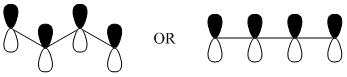Figure $$\PageIndex{12}$$. Representation of the four p-orbitals in 1,3-butadiene.

## Using representations of the p-orbitals in which the dark color indicates the positive region of the wave function and a light color indicates the negative region of the wave function, draw all of the possible ways in which the wave functions of the four p-orbitals can overlap with each other.

The four pictures in Figure $$\PageIndex{13}$$ represent the possible alignments of the signs of the wave functions in 1,3-butadiene. In Figure $$\PageIndex{13}$$a, all four p-orbitals constructively overlap with each other. In Figure $$\PageIndex{13}$$b, two adjacent pairs of p-orbitals constructively overlap with each other. In Figure $$\PageIndex{13}$$c, only the pair of p-orbitals in the center has constructive overlap. In Figure $$\PageIndex{14}$$d, there is no constructive overlap and only destructive overlap occurs.Figure $$\PageIndex{13}$$. Possible alignments of the signs of the wave functions of the p-orbitals in 1,3-butadiene.

Rank these from high to low energy.

The orbital in which all four p-orbitals overlap would be the lowest in energy (Figure $$\PageIndex{14}$$). The next has two regions of overlap. The third has only one region of overlap and the highest energy orbital has no regions of overlap. Because there are four electrons to put in the orbitals (one from each of the contributing p-orbitals), the bottom two orbitals are filled and the top two are empty.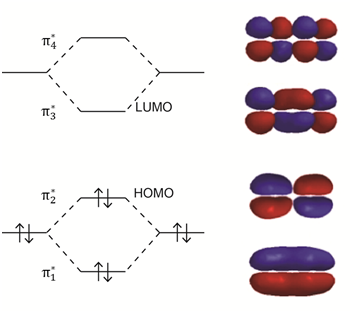Figure $$\PageIndex{14}$$. Energy level diagram and representation of the $$\pi$$- and $$\pi$$*-molecular orbitals in 1,3-butadiene.

The lowest energy HOMO to LUMO transition will be lower than observed in 1-butene. The UV/VIS spectrum of 1,3-butadiene is shown in Figure $$\PageIndex{15}$$. In this case, the $$\lambda$$max value is at about 292 nm, a significant difference from the value of 176 nm in 1-butene. The effect of increasing conjugation is to shift the spectrum toward longer wavelength (lower frequency, lower energy) absorptions.Figure $$\PageIndex{15}$$. Ultraviolet absorption spectrum of 1,3-butadiene.

Another comparative set of conjugated systems occurs with fused ring polycyclic aromatic hydrocarbons such as naphthalene, anthracene and pentacene.The spectra in Figure $$\PageIndex{16}$$ are for benzene, naphthalene, anthracene and pentacene. Note that as more rings and more conjugation are added, the spectrum shifts further toward and into the visible region of the spectrum.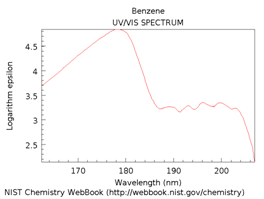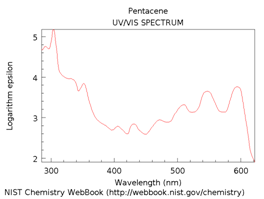Figure $$\PageIndex{16}$$. UV/VIS absorption spectra of benzene, naphthalene, anthracene and pentacene.

This page titled 2.2: Effect of Conjugation is shared under a CC BY-NC 4.0 license and was authored, remixed, and/or curated by Thomas Wenzel via source content that was edited to the style and standards of the LibreTexts platform; a detailed edit history is available upon request.